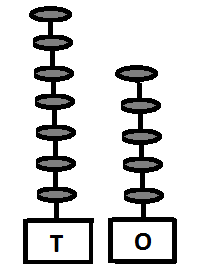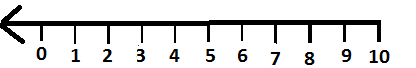1. Addition means to add.  Suppose Ram eats two toffees on Sunday and six toffees on Monday, then total toffees he has eaten on Sunday and Monday is 2 + 6 = 8

2. These are 2 arrows3. If one more arrow is added it is three arrows = 3 arrows4. If two more arrows are added , there are four arrows 2 + 3 = 55. If six more arrows are added there are eight arrows, 2 + 6 = 8 arrows6. Two marbles and three marbles make five marbles = 2 + 3 = 5 marbles = 4 balls  + 3 balls = 7 balls7. 5 + 4 or five plus four is equal to = 9

8. When we add 8 in 20 we get 28. It has 2 tens and 8 ones. 2 X 10 = 20 + 8 ones.

9. Observe these numbers - 35, 67, 24, 82, 76, 49.

10. When we arrange these from lowest to highest it is 24, 35, 49, 67, 76, 82. This arrangement is ascending order

11. When we arrange from highest to lowest the arrangement is 82, 76, 67, 49, 35, 24. This arrangement is descending order.

12. If we add zero to a number we get the same number. For instance 0 + 46 = 46.13. In 75, there are seven tens which is equal to 7 X 10 = 70 and 5 ones which is equal to 5 X 1 = 5, hence it is 70 + 5 = 75

14. If you have 10 pencils and you purchase 6 more pencils, then you would have 16 pencils  ( 10 + 6 )

15. You have 80 erasers and you have 9 more marbles than the number of erasers, then you have 80 + 9 = 89 marbles.16. When we add 3 to 5 on the number line it would be at 8.